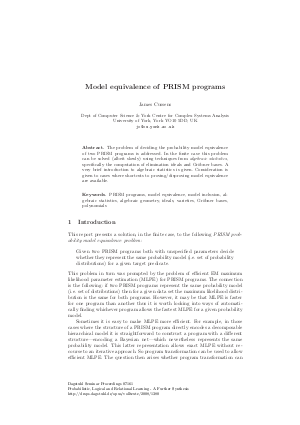Document# Model equivalence of PRISM programs

### Author James Cussens## File

DagSemProc.07161.7.pdf
• Filesize: 192 kB
• 21 pages

## Cite As

James Cussens. Model equivalence of PRISM programs. In Probabilistic, Logical and Relational Learning - A Further Synthesis. Dagstuhl Seminar Proceedings, Volume 7161, pp. 1-21, Schloss Dagstuhl - Leibniz-Zentrum für Informatik (2008)
https://doi.org/10.4230/DagSemProc.07161.7

## Abstract

The problem of deciding the probability model equivalence of two PRISM programs is addressed. In the finite case this problem can be solved (albeit slowly) using techniques from emph{algebraic statistics}, specifically the computation of elimination ideals and Gr"{o}bner bases. A very brief introduction to algebraic statistics is given. Consideration is given to cases where shortcuts to proving/disproving model equivalence are available.
##### Keywords
• PRISM programs
• model equivalence
• model inclusion
• algebraic statistics
• algebraic geometry
• ideals
• varieties
• Gr"{o}bner bases
• polynomials

## Metrics

• Access Statistics
• Total Accesses (updated on a weekly basis)
0